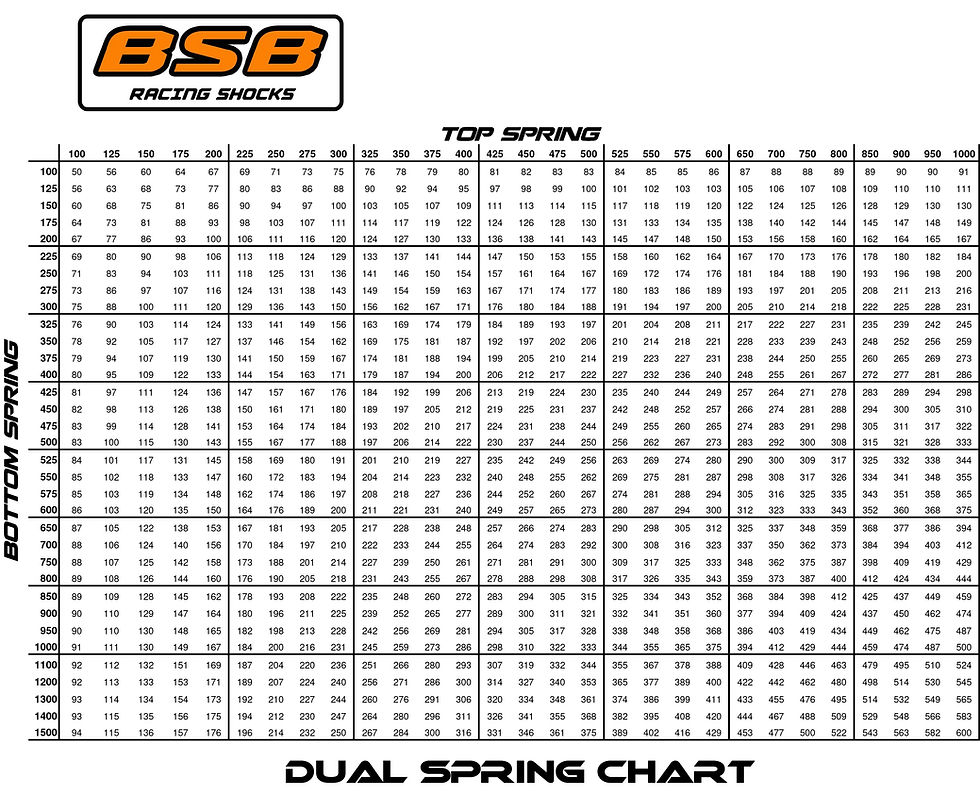top of pageSearch

# Stack Spring Chart

Updated: Aug 3, 2022

Well in this world we stack springs more and more and this chart will help you get to the answer faster. If your old school and want to do the math well here that is too.

Rate x Rate = A

Rate + Rate = B

A / B = C and C is the new rate.

700 x 300 = 210000 , 700 + 300 = 1000 210000 / 1000 = 210 the stack rate is 210 lbs